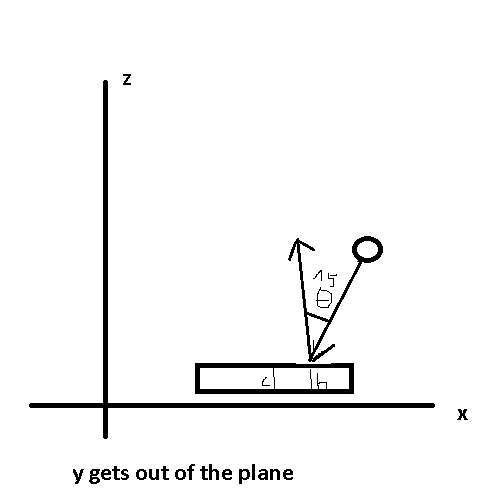# change ball direction when hitting paddle

hi, i wanted to change the direction from the ball depending where the ball hits on my paddle.

i got it to work kinda but it is affecting the speed from the ball.

here is my code :

``````function OnCollisionEnter(ballCollision : Collision)
{

var p1PosHit : float = ballHit.transform.position.y - paddleP1.transform.position.y;
var calculateY : float = rigidbody.velocity.y + p1PosHit;

//player 1 on collision
if(ballCollision.gameObject.name == "Player1" )
{

rigidbody.velocity = Vector3(rigidbody.velocity.x,calculateY,0);
}
``````

it is a pong clone btw and 2D on x and y (z = 0)
and there is no gravity or drag

If you want to bounce your ball 15 degrees, that takes about 1 line.

``````function OnCollisionEnter(other:Collision){
if(other.gameObject.tag == "Cube")
vel = Quaternion.AngleAxis(-15, Vector3.up) * -vel;
}
``````

This assumes your game is in the x and z direction (flat on the ground) and the y-axis is going out of the screen.

Your vel is the velocity of your ball, Quaternion.AngleAxis takes how much it is supposed to rotate, and the vector on which it should rotate. In our case, -15 and the up axis (y-axis, same things different names). Now we need to invert the ball velocity so we multiply by -vel.

I will not go into Quaternion as it is complex number. Just know that it fixes some eulerAngle issues.

Now your problem is that it will always bounce 15 degrees in the same direction.

But considering that your pad is along the x-axis (See pic) (meaning it only moves its position.x) if you subtract the contact position-x with the position.x of the pad, you can know where the ball hit. If the value is <0 then you are on the left you apply -15 and the other way around.

See on the pics, if h is greater than c then you are on the right side. You apply 15 or -15 depending on what you want (the pics shows -15).

Well, all in all I guess that is what you needed now you just use it the way you want.